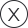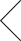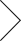Light Gold Bridesmaid Dress | bsidetest• # Light Gold

##### \$3.00• # Light Gold

• ##### \$3.00
• ###### AVAILABLE COLORS
second condition
• ###### Size
View Aura size chart
• ###### Estimated Week of Delivery: now = new Date(); fourteenweeksfromnow = new Date(now.getTime() + (98 * 24 * 60 * 60 * 1000)); elevenweeksfromnow = new Date(now.getTime() + (77 * 24 * 60 * 60 * 1000)); twelveweeksfromnow = new Date(now.getTime() + (84 * 24 * 60 * 60 * 1000)); tenweeksfromnow = new Date(now.getTime() + (70 * 24 * 60 * 60 * 1000)); sixteenweeksfromnow = new Date(now.getTime() + (112 * 24 * 60 * 60 * 1000)); seventeenweeksfromnow = new Date(now.getTime() + (119 * 24 * 60 * 60 * 1000)); sixweeksfromnow = new Date(now.getTime() + (42 * 24 * 60 * 60 * 1000)); sevenweeksfromnow = new Date(now.getTime() + (49 * 24 * 60 * 60 * 1000)); twoweeksfromnow = new Date(now.getTime() + (14 * 24 * 60 * 60 * 1000)); eighteenweeksfromnow = new Date(now.getTime() + (126 * 24 * 60 * 60 * 1000)); eleven_n_halfweeksfromnow = new Date(now.getTime() + (80 * 24 * 60 * 60 * 1000)); thirteenweeksfromnow = new Date(now.getTime() + (91 * 24 * 60 * 60 * 1000)); fifteenweeksfromnow = new Date(now.getTime() + (105 * 24 * 60 * 60 * 1000)); nineweeksfromnow = new Date(now.getTime() + (63 * 24 * 60 * 60 * 1000)); nine_n_halfweeksfromnow = new Date(now.getTime() + (68 * 24 * 60 * 60 * 1000)); threeweeksfromnow = new Date(now.getTime() + (21 * 24 * 60 * 60 * 1000)); threeweeksnhalffromnow = new Date(now.getTime() + (56 * 24 * 60 * 60 * 1000)); nineteenweeksfromnow = new Date(now.getTime() + (133 * 24 * 60 * 60 * 1000)); fourweeknhalffromnow = new Date(now.getTime() + (32 * 24 * 60 * 60 * 1000)); months = new Array("January ","February ","March ","April ","May ","June ","July ","August ","September ","October ","November ","December "); document.write(months[elevenweeksfromnow.getMonth()] + elevenweeksfromnow.getDate() ); // var DeliveryDate = elevenweeksfromnow.getMonth()+1+"/"+elevenweeksfromnow.getDate()+"/"+elevenweeksfromnow.getFullYear(); var DeliveryDate = months[elevenweeksfromnow.getMonth()] + elevenweeksfromnow.getDate();
•• ###### Do you need it faster?Call us today!

773-234-1787

Customer Reviews